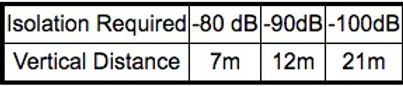## 1. Measuring the Donor Signal Strength:

The minimum required donor BTS signal strength received at the repeater sight is a dominant requirement.

The following example represents the option to measure the signal strength using a cellular phone set to " Engineering Mode". Measurement is done on the tower where the donor antenna will be mounted.

## a. Donor signal strength for F1/F2 repeaters

An F1/F2 repeater has output power of +43dBm max (20W). Since its max gain (assuming the isolation between the repeater antennas is infinite) is +115dB, the minimum signal strength at the donor input of the repeater should be -72dBm (+43-115).

Since the repeater antenna looking at the donor has +17dbi gain and the cellular phone has antenna with 0dBi gain, the minimum signal reading at the cellular phone should be -89dBm

## b. Donor signal strength for RF repeaters

An RF repeater has output power of +43dBm max (20W). Since its max gain (assuming the isolation between the repeater antennas is infinite) is +90dB,

the minimum signal strength at the donor input of the repeater should be -47dBm (+43-90).

Since the repeater antenna looking at the donor has +17dbi gain and the cellular phone has antenna with 0dBi gain, the minimum signal reading at the cellular phone should be -64dBm

## 2. Setting the Repeater Antennas for Adequate Isolation

With RF repeaters, for which the input signal frequency is also the output signal frequency, the repeater will not oscillate only if the isolation between its donor and distribution antennas will be at least 10dB higher than the gain of the repeater.

With RF repeaters of +90dB gain, the isolation between the antennas should be at least 100dB. This isolation should be measured before repeater installation.

If measurement is impossible, use this very simplistic equation to calculate the vertical distance required between the two repeater's antennas to meet the Isolation condition.

Isolation[dB]=28+40log[S/λ]

Where S is the vertical distance between the antennas and λ is the wavelength.

For 900MHz GSM band the following values are applicable:With F1/F2 repeaters the antenna isolation is less critical and can be settled for -80dB

If the required gain is lower than the repeaters maximum available gain, the isolation between the antennas can be lower and so can the distance between these antennas.

It is thus recommended to use a very high gain antenna for the donor BTS reception so the signal will be maximum.

If the BTS signal is received at a reasonable level, your choice should be to put the distribution antenna of the repeater at the top of the tower and the donor antenna of the repeater S meters below .

This way the repeater can cover a larger area in a better way. If the donor BTS signal received is too low and the donor antenna has to be mounted on top of the tower for reasonable signal reception, the distribution antenna will be mounted S meters below the top and the coverage will be degraded.